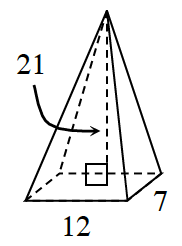### Home > INT2 > Chapter 12 > Lesson 12.1.2 > Problem12-23

12-23.

Multiple Choice: Calculate the volume of the rectangle-based pyramid at right.

1. $84 \; \text{units}^3$

1. $588 \; \text{units}^3$

1. $882 \; \text{units}^3$

1. $1764 \; \text{units}^3$

1. none of theseB